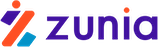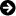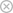Tuyển sinh 2023 dành cho Gen-Z# Sorting and Searching Algorithms: A Cookbook

Chia sẻ: Ledung Ledung | Ngày: | Loại File: PDF | Số trang:36134
lượt xem
13

The first section introduces basic data structures and notation. The next section presents several sorting algorithms. This is followed by techniques for implementing dictionaries, structures that allow efficient search, insert, and delete operations. The last section illustrates algorithms that sort data and implement dictionaries for very large files. Source code for each algorithm, in ANSI C, is available at the site listed below

Chủ đề:

## Nội dung Text: Sorting and Searching Algorithms: A Cookbook

1. Sorting and Searching Algorithms: A Cookbook Thomas Niemann
2. Preface This is a collection of algorithms for sorting and searching. Descriptions are brief and intuitive, with just enough theory thrown in to make you nervous. I assume you know C, and that you are familiar with concepts such as arrays and pointers. The first section introduces basic data structures and notation. The next section presents several sorting algorithms. This is followed by techniques for implementing dictionaries, structures that allow efficient search, insert, and delete operations. The last section illustrates algorithms that sort data and implement dictionaries for very large files. Source code for each algorithm, in ANSI C, is available at the site listed below. Permission to reproduce this document, in whole or in part, is given provided the original web site listed below is referenced, and no additional restrictions apply. Source code, when part of a software project, may be used freely without reference to the author. THOMAS NIEMANN Portland, Oregon email: thomasn@jps.net home: http://members.xoom.com/thomasn/s_man.htm By the same author: A Guide to Lex and Yacc, at http://members.xoom.com/thomasn/y_man.htm. -2-
3. CONTENTS 1. INTRODUCTION 4 2. SORTING 8 2.1 Insertion Sort 8 2.2 Shell Sort 10 2.3 Quicksort 11 2.4 Comparison 14 3. DICTIONARIES 15 3.1 Hash Tables 15 3.2 Binary Search Trees 19 3.3 Red-Black Trees 21 3.4 Skip Lists 25 3.5 Comparison 26 4. VERY LARGE FILES 29 4.1 External Sorting 29 4.2 B-Trees 32 5. BIBLIOGRAPHY 36 -3-
4. 1. Introduction Arrays and linked lists are two basic data structures used to store information. We may wish to search, insert or delete records in a database based on a key value. This section examines the performance of these operations on arrays and linked lists. Arrays Figure 1-1 shows an array, seven elements long, containing numeric values. To search the array sequentially, we may use the algorithm in Figure 1-2. The maximum number of comparisons is 7, and occurs when the key we are searching for is in A. 0 4 Lb 1 7 2 16 3 20 M 4 37 5 38 6 43 Ub Figure 1-1: An Array int function SequentialSearch (Array A , int Lb , int Ub , int Key ); begin for i = Lb to Ub do if A [ i ] = Key then return i ; return –1; end; Figure 1-2: Sequential Search -4-
5. int function BinarySearch (Array A , int Lb , int Ub , int Key ); begin do forever M = ( Lb + Ub )/2; if ( Key < A[M]) then Ub = M – 1; else if (Key > A[M]) then Lb = M + 1; else return M ; if (Lb > Ub) then return –1; end; Figure 1-3: Binary Search If the data is sorted, a binary search may be done (Figure 1-3). Variables Lb and Ub keep track of the lower bound and upper bound of the array, respectively. We begin by examining the middle element of the array. If the key we are searching for is less than the middle element, then it must reside in the top half of the array. Thus, we set Ub to (M – 1). This restricts our next iteration through the loop to the top half of the array. In this way, each iteration halves the size of the array to be searched. For example, the first iteration will leave 3 items to test. After the second iteration, there will be one item left to test. Therefore it takes only three iterations to find any number. This is a powerful method. Given an array of 1023 elements, we can narrow the search to 511 elements in one comparison. After another comparison, and we’re looking at only 255 elements. In fact, we can search the entire array in only 10 comparisons. In addition to searching, we may wish to insert or delete entries. Unfortunately, an array is not a good arrangement for these operations. For example, to insert the number 18 in Figure 1-1, we would need to shift A…A down by one slot. Then we could copy number 18 into A. A similar problem arises when deleting numbers. To improve the efficiency of insert and delete operations, linked lists may be used. -5-
6. Linked Lists X 18 P # 4 7 16 20 37 38 43 Figure 1-4: A Linked List In Figure 1-4 we have the same values stored in a linked list. Assuming pointers X and P, as shown in the figure, value 18 may be inserted as follows: X->Next = P->Next; P->Next = X; Insertion and deletion operations are very efficient using linked lists. You may be wondering how pointer P was set in the first place. Well, we had to do a sequential search to find the insertion point X. Although we improved our performance for insertion/deletion, it was done at the expense of search time. Timing Estimates Several methods may be used to compare the performance of algorithms. One way is simply to run several tests for each algorithm and compare the timings. Another way is to estimate the time required. For example, we may state that search time is O(n) (big-oh of n). This means that search time, for large n, is proportional to the number of items n in the list. Consequently, we would expect search time to triple if our list increased in size by a factor of three. The big-O notation does not describe the exact time that an algorithm takes, but only indicates an upper bound on execution time within a constant factor. If an algorithm takes O(n2) time, then execution time grows no worse than the square of the size of the list. -6-
7. n lg n n lg n n 1.25 n2 1 0 0 1 1 16 4 64 32 256 256 8 2,048 1,024 65,536 4,096 12 49,152 32,768 16,777,216 65,536 16 1,048,565 1,048,476 4,294,967,296 1,048,476 20 20,969,520 33,554,432 1,099,301,922,576 16,775,616 24 402,614,784 1,073,613,825 281,421,292,179,456 Table 1-1: Growth Rates Table 1-1 illustrates growth rates for various functions. A growth rate of O(lg n) occurs for algorithms similar to the binary search. The lg (logarithm, base 2) function increases by one when n is doubled. Recall that we can search twice as many items with one more comparison in the binary search. Thus the binary search is a O(lg n) algorithm. If the values in Table 1-1 represented microseconds, then a O(lg n) algorithm may take 20 microseconds to process 1,048,476 items, a O(n1.25) algorithm might take 33 seconds, and a O(n2) algorithm might take up to 12 days! In the following chapters a timing estimate for each algorithm, using big-O notation, will be included. For a more formal derivation of these formulas you may wish to consult the references. Summary As we have seen, sorted arrays may be searched efficiently using a binary search. However, we must have a sorted array to start with. In the next section various ways to sort arrays will be examined. It turns out that this is computationally expensive, and considerable research has been done to make sorting algorithms as efficient as possible. Linked lists improved the efficiency of insert and delete operations, but searches were sequential and time-consuming. Algorithms exist that do all three operations efficiently, and they will be the discussed in the section on dictionaries. -7-
8. 2. Sorting Several algorithms are presented, including insertion sort, shell sort, and quicksort. Sorting by insertion is the simplest method, and doesn’t require any additional storage. Shell sort is a simple modification that improves performance significantly. Probably the most efficient and popular method is quicksort, and is the method of choice for large arrays. 2.1 Insertion Sort One of the simplest methods to sort an array is an insertion sort. An example of an insertion sort occurs in everyday life while playing cards. To sort the cards in your hand you extract a card, shift the remaining cards, and then insert the extracted card in the correct place. This process is repeated until all the cards are in the correct sequence. Both average and worst-case time is O(n2). For further reading, consult Knuth . -8-
9. Theory Starting near the top of the array in Figure 2-1(a), we extract the 3. Then the above elements are shifted down until we find the correct place to insert the 3. This process repeats in Figure 2-1(b) with the next number. Finally, in Figure 2-1(c), we complete the sort by inserting 2 in the correct place. 4 4 3 3 4 4 D
10. 1 1 1 1 2 2 2 2 3 3 1 4 4 3 3 E
11. 1 4 4 2 2 2 2 1 1 1 1 3 3 2 F
12. 4 4 3 3 2 4 4 Figure 2-1: Insertion Sort Assuming there are n elements in the array, we must index through n – 1 entries. For each entry, we may need to examine and shift up to n – 1 other entries, resulting in a O(n2) algorithm. The insertion sort is an in-place sort. That is, we sort the array in-place. No extra memory is required. The insertion sort is also a stable sort. Stable sorts retain the original ordering of keys when identical keys are present in the input data. Implementation Source for the insertion sort algorithm may be found in file ins.c. Typedef T and comparison operator compGT should be altered to reflect the data stored in the table. -9-
13. 2.2 Shell Sort Shell sort, developed by Donald L. Shell, is a non-stable in-place sort. Shell sort improves on the efficiency of insertion sort by quickly shifting values to their destination. Average sort time is O(n1.25), while worst-case time is O(n1.5). For further reading, consult Knuth . Theory In Figure 2-2(a) we have an example of sorting by insertion. First we extract 1, shift 3 and 5 down one slot, and then insert the 1, for a count of 2 shifts. In the next frame, two shifts are required before we can insert the 2. The process continues until the last frame, where a total of 2 + 2 + 1 = 5 shifts have been made. In Figure 2-2(b) an example of shell sort is illustrated. We begin by doing an insertion sort using a spacing of two. In the first frame we examine numbers 3-1. Extracting 1, we shift 3 down one slot for a shift count of 1. Next we examine numbers 5-2. We extract 2, shift 5 down, and then insert 2. After sorting with a spacing of two, a final pass is made with a spacing of one. This is simply the traditional insertion sort. The total shift count using shell sort is 1+1+1 = 3. By using an initial spacing larger than one, we were able to quickly shift values to their proper destination. 2s 2s 1s 3 1 1 1 5 3 2 2 D
14. 1 5 3 3 2 2 5 4 4 4 4 5 1s 1s 1s 3 1 1 1 5 5 2 2 E
15. 1 3 3 3 2 2 5 4 4 4 4 5 Figure 2-2: Shell Sort Various spacings may be used to implement shell sort. Typically the array is sorted with a large spacing, the spacing reduced, and the array sorted again. On the final sort, spacing is one. Although the shell sort is easy to comprehend, formal analysis is difficult. In particular, optimal spacing values elude theoreticians. Knuth has experimented with several values and recommends that spacing h for an array of size N be based on the following formula: Let h1 = 1, hs +1 = 3hs + 1, and stop with ht when ht + 2 ≥ N - 10 -
16. Thus, values of h are computed as follows: h1 = 1 h2 = (3 × 1) + 1 = 4 h3 = (3 × 4) + 1 = 13 h4 = (3 × 13) + 1 = 40 h5 = (3 × 40) + 1 = 121 To sort 100 items we first find hs such that hs ≥ 100. For 100 items, h5 is selected. Our final value (ht) is two steps lower, or h3. Therefore our sequence of h values will be 13-4-1. Once the initial h value has been determined, subsequent values may be calculated using the formula hs −1 = hs / 3 Implementation Source for the shell sort algorithm may be found in file shl.c. Typedef T and comparison operator compGT should be altered to reflect the data stored in the array. The central portion of the algorithm is an insertion sort with a spacing of h. 2.3 Quicksort Although the shell sort algorithm is significantly better than insertion sort, there is still room for improvement. One of the most popular sorting algorithms is quicksort. Quicksort executes in O(n lg n) on average, and O(n2) in the worst-case. However, with proper precautions, worst-case behavior is very unlikely. Quicksort is a non-stable sort. It is not an in-place sort as stack space is required. For further reading, consult Cormen . Theory The quicksort algorithm works by partitioning the array to be sorted, then recursively sorting each partition. In Partition (Figure 2-3), one of the array elements is selected as a pivot value. Values smaller than the pivot value are placed to the left of the pivot, while larger values are placed to the right. - 11 -
17. int function Partition (Array A, int Lb, int Ub); begin select a pivot from A[Lb]…A[Ub]; reorder A[Lb]…A[Ub] such that: all values to the left of the pivot are ≤ pivot all values to the right of the pivot are ≥ pivot return pivot position; end; procedure QuickSort (Array A, int Lb, int Ub); begin if Lb < Ub then M = Partition (A, Lb, Ub); QuickSort (A, Lb, M – 1); QuickSort (A, M + 1, Ub); end; Figure 2-3: Quicksort Algorithm In Figure 2-4(a), the pivot selected is 3. Indices are run starting at both ends of the array. One index starts on the left and selects an element that is larger than the pivot, while another index starts on the right and selects an element that is smaller than the pivot. In this case, numbers 4 and 1 are selected. These elements are then exchanged, as is shown in Figure 2-4(b). This process repeats until all elements to the left of the pivot are ≤ the pivot, and all items to the right of the pivot are ≥ the pivot. QuickSort recursively sorts the two sub-arrays, resulting in the array shown in Figure 2-4(c). Lb Ub D
18. 4 2 3 5 1 SLYRW Lb M Lb E
19. 1 2 3 5 4 F
20. 1 2 3 4 5 Figure 2-4: Quicksort Example As the process proceeds, it may be necessary to move the pivot so that correct ordering is maintained. In this manner, QuickSort succeeds in sorting the array. If we’re lucky the pivot selected will be the median of all values, equally dividing the array. For a moment, let’s assume - 12 -